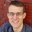Question-and-Answer Resource for the Building Energy Modeling Community
Get started with the Help page

# Design flow rate and pressure difference (50Pa?)

I'm trying to define in OpenStudio Space Infiltration Design Flow rate, by using a design flow rate parameters of a buildings similar to my study cases. My reference data have the results with 50Pa of pressure difference. I read the engineering reference and don't see any limit to the pressure use for that. (For the case of Effective Leakage area, it is well defined that should be 4Pa) Can i use a flow rate of 50Pa for the simulation? I hope anyone can help me,

edit retag close merge delete

Sort by » oldest newest most votedThe OpenStudio Space Infiltration Design Flow rate input is assumed to be at natural pressure. So you need to convert the infiltration rate at 50 Pa to the rate at natural pressure (~4 Pa).

See the answer to "how to set air infiltration under 50 pa pressure" on the forum. There is a table for residential projects to convert ACH50 to ACHnat.

Otherwise, you can calculate it from Q_delta_P = C*(delta_P)^n, where Q is the infiltration rate at pressure differential delta_P, n = 0.65, and C is the flow coefficient. Given your known infiltration Q at 50 Pa, calculate C. Then change delta_P to natural pressure (~4 Pa) and calculate Q.

more